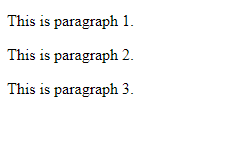# How to loop through HTML elements without using forEach() loop in JavaScript ?

In this article, we will learn how to loop through HTML elements without using the forEach() method.

This can be done in the following ways:

Table of Content

## Approach 1: Using the for loop

The HTML elements can be iterated by using the regular JavaScript for loop. The number of elements to be iterated can be found using the length property. The for loop has three parts, initialization, condition expression, and increment/decrement expression. Each of the items can be accessed by using square brackets with their respective index.

### Syntax:

`for (let i = 0; i < elements.length; i++) {    console.log(elements[i]);}`

Example: In this example, we will see the use of the for loop.

## HTML

 `` `<``html` `lang``=``"en"``>`   `<``head``>` `    ``<``title``>` `        ``How to loop through HTML elements` `        ``without using forEach() loop` `        ``in JavaScript ?` `    ``` ``   `<``body``>` `    ``` `    ``<``p``>This is paragraph 1.` `    ``<``p``>This is paragraph 2.` `    ``<``p``>This is paragraph 3.`   `    ``<``script` `type``=``"text/javascript"``>` `        ``// Get the elements to be iterated` `        ``let htmlElements =` `            ``document.getElementsByTagName("p");`   `        ``// Use a regular for-loop` `        ``for (let i = 0; i < ``htmlElements.length``; i++) {`   `            ``// Print the current element` `            ``console.log(htmlElements[i]);` `        ``}` `    ``` ``   ``

Output:Output on the browser

## Approach 2: Using the While loop

The HTML elements can be iterated by using a regular JavaScript while loop. The number of elements can be found using the length property. A temporary value is used to keep track of the current iteration by checking it in the condition expression. Each of the items can then be accessed by using square brackets with their respective index.

### Syntax:

`let i = 0;while(i < elements.length) {    console.log(elements[i]);    i++;}`

Example: In this example, we will see the use of Using the While loop.

## HTML

 `` `<``html` `lang``=``"en"``>`   `<``head``>` `    ``<``title``>` `        ``How to loop through HTML` `        ``elements without using` `        ``forEach() loop in JavaScript ?` `    ``` ``   `<``body``>` `    ``` `    ``<``p``>This is paragraph 1.` `    ``<``p``>This is paragraph 2.` `    ``<``p``>This is paragraph 3.`   `    ``<``script` `type``=``"text/javascript"``>` `        ``// Get the elements to be iterated` `        ``let htmlElements =` `            ``document.getElementsByTagName("p");`   `        ``// Define a variable to keep track` `        ``// of the current iteration` `        ``let i = 0;`   `        ``// Check if the current value` `        ``// is lesser than total elements` `        ``while (i < ``htmlElements.length``) {`   `            ``// Print the current element` `            ``console.log(htmlElements[i]);`   `            ``// Increment the value` `            ``i++;` `        ``}` `    ``` ``   ``

Output:Output

## Approach 3:Using the ‘for…..of’ statement

The for…of statement can be used to loop over values of an iterable object. It includes objects like an Array, Map, Set, or HTML elements. A temporary variable holds the current value during the execution of the loop, which can then be used in the body of the loop.

### Syntax:

`for (element of elements) {    console.log(element);}`

Example: In this example, we will see the use of Using the ‘for…..of’ statement.

## HTML

 `` `<``html` `lang``=``"en"``>`   `<``head``>` `    ``<``title``>` `        ``How to loop through HTML` `        ``elements without using` `        ``forEach() loop in JavaScript ?` `    ``` ``   `<``body``>` `    ``` `    ``<``p``>This is paragraph 1.` `    ``<``p``>This is paragraph 2.` `    ``<``p``>This is paragraph 3.`   `    ``<``script` `type``=``"text/javascript"``>` `        ``// Get the elements to be iterated` `        ``let HTMLelements =` `            ``document.getElementsByTagName("p");`   `        ``// Use the for...of statement to get` `        ``// the values` `        ``for (elem of HTMLelements) {`   `            ``// Print the current element` `            ``console.log(elem);` `        ``}` `    ``` ``   ``

Output:Output

Whether you're preparing for your first job interview or aiming to upskill in this ever-evolving tech landscape, GeeksforGeeks Courses are your key to success. We provide top-quality content at affordable prices, all geared towards accelerating your growth in a time-bound manner. Join the millions we've already empowered, and we're here to do the same for you. Don't miss out - check it out now!

Previous
Next